# smoothdata

Smooth noisy data

## Syntax

``B = smoothdata(A)``
``B = smoothdata(A,dim)``
``B = smoothdata(___,method)``
``B = smoothdata(___,method,window)``
``B = smoothdata(___,nanflag)``
``B = smoothdata(___,Name,Value)``
``````[B,winsize] = smoothdata(___)``````

## Description

example

````B = smoothdata(A)` smooths entries of `A` using a moving average. `smoothdata` determines the moving window size from the entries in `A`. The window slides down the length of the vector, computing an average over the elements within each window. If `A` is a matrix, then `smoothdata` computes the moving average down each column of `A`.If `A` is a multidimensional array, then `smoothdata` operates along the first dimension of `A` whose size does not equal 1.If `A` is a table or timetable with numeric variables, then `smoothdata` operates on each variable of `A` separately. ```

example

````B = smoothdata(A,dim)` specifies the dimension of `A` to operate along. For example, if `A` is a matrix, then `smoothdata(A,2)` smooths the data in each row of `A`.```

example

````B = smoothdata(___,method)` specifies the smoothing method for either of the previous syntaxes. For example, `smoothdata(A,"sgolay")` uses a Savitzky-Golay filter to smooth the data in `A`.```

example

````B = smoothdata(___,method,window)` specifies the smoothing method window size. For example, `smoothdata(A,"movmedian",5)` smooths the data in `A` by taking the median over a five-element sliding window.```

example

````B = smoothdata(___,nanflag)` specifies whether to omit or include `NaN` values in `A`. For example, `smoothdata(A,"includenan")` includes all `NaN` values when smoothing. By default, `smoothdata` ignores `NaN` values.```

example

````B = smoothdata(___,Name,Value)` specifies additional parameters for smoothing using one or more name-value arguments. For example, if `t` is a vector of time values, then `smoothdata(A,"SamplePoints",t)` smooths the data in `A` relative to the times in `t`.```

example

``````[B,winsize] = smoothdata(___)``` also returns the moving window size.```

## Examples

collapse all

Create a vector containing noisy data, and smooth the data with a moving average.

```x = 1:100; rng(0,"twister") A = cos(2*pi*0.05*x+2*pi*rand) + 0.5*randn(1,100); B = smoothdata(A);```

Plot the original and smoothed data.

```plot(x,A) hold on plot(x,B) legend("Input Data","Smoothed Data")```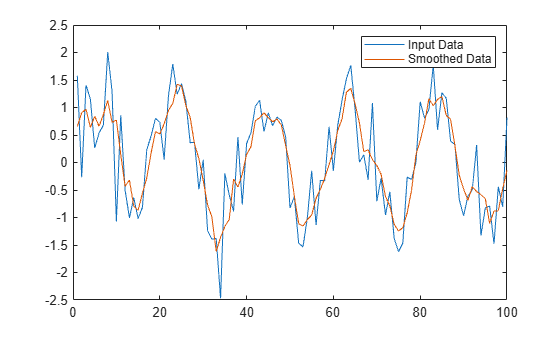Create a matrix whose rows represent three noisy signals. Smooth the three signals using a moving average, and plot the smoothed data.

```x = 1:100; rng(0,"twister") s1 = cos(2*pi*0.03*x+2*pi*rand) + 0.5*randn(1,100); s2 = cos(2*pi*0.04*x+2*pi*rand) + 0.4*randn(1,100) + 5; s3 = cos(2*pi*0.05*x+2*pi*rand) + 0.3*randn(1,100) - 5; A = [s1; s2; s3]; B = smoothdata(A,2); plot(x,B(1,:)) hold on plot(x,B(2,:)) plot(x,B(3,:)) legend("s1","s2","s3")```Smooth a vector of noisy data with a Gaussian-weighted moving average filter. Display the window size used by the filter.

```x = 1:100; rng(0,"twister") A = cos(2*pi*0.05*x+2*pi*rand) + 0.5*randn(1,100); [B,winsize] = smoothdata(A,"gaussian"); winsize```
```winsize = 4 ```

Smooth the original data with a larger window containing 20 elements. Plot the smoothed data for both window sizes.

```C = smoothdata(A,"gaussian",20); plot(x,B) hold on plot(x,C) legend("Small Window","Large Window")```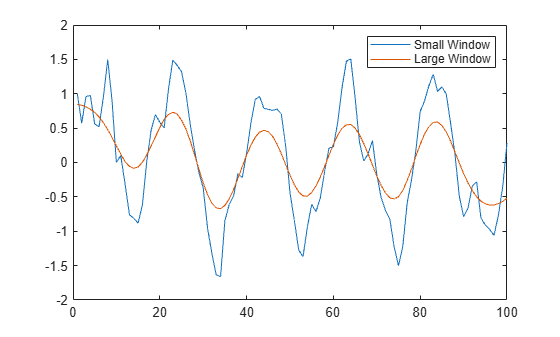Create a noisy vector containing `NaN` values, and smooth the data ignoring `NaN` values.

```rng(0,"twister") A = [NaN randn(1,48) NaN randn(1,49) NaN]; B = smoothdata(A);```

Smooth the data including `NaN` values. The average in a window containing any `NaN` value is `NaN`.

`C = smoothdata(A,"includenan");`

Plot the smoothed data in `B` and `C`.

```plot(1:100,B,"-o") hold on plot(1:100,C,"-x") legend("Ignore Missing","Include Missing")```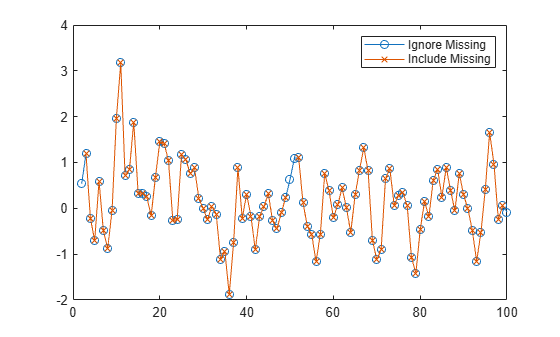Create a vector of noisy data that corresponds to a time vector `t`. Smooth the data relative to the times in `t`, and plot the original data and the smoothed data.

```x = 1:100; rng(0,"twister") A = cos(2*pi*0.05*x+2*pi*rand) + 0.5*randn(1,100); t = datetime(2017,1,1,0,0,0) + hours(0:99); B = smoothdata(A,"SamplePoints",t); plot(t,A) hold on plot(t,B) legend("Input Data","Smoothed Data")```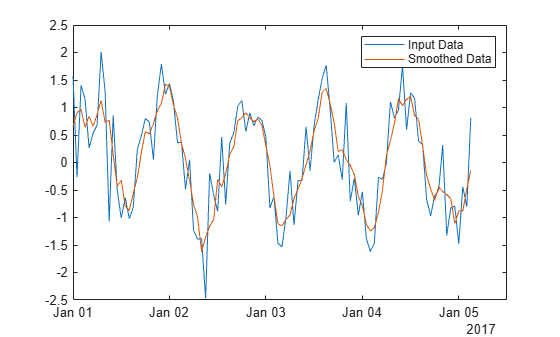## Input Arguments

collapse all

Input data, specified as a vector, matrix, multidimensional array, table, or timetable. If `A` is a table or timetable, then either the variables must be numeric, or you must use the `DataVariables` name-value argument to list numeric variables explicitly. Specifying variables is useful when you are working with a table that also contains nonnumeric variables.

Data Types: `double` | `single` | `int8` | `int16` | `int32` | `int64` | `uint8` | `uint16` | `uint32` | `uint64` | `logical` | `table` | `timetable`

Complex Number Support: Yes

Operating dimension, specified as a positive integer scalar. If no value is specified, then the default is the first array dimension whose size does not equal 1.

Consider an `m`-by-`n` input matrix, `A`:

• `smoothdata(A,1)` smooths the data in each column of `A` and returns an `m`-by-`n` matrix.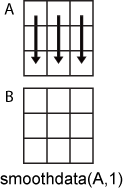• `smoothdata(A,2)` smooths the data in row of `A` and returns an `m`-by-`n` matrix.For table or timetable input data, `dim` is not supported and operation is along each table or timetable variable separately.

Smoothing method, specified as one of these values:

• `"movmean"` — Average over each window of `A`. This method is useful for reducing periodic trends in data.

• `"movmedian"` — Median over each window of `A`. This method is useful for reducing periodic trends in data when outliers are present.

• `"gaussian"` — Gaussian-weighted average over each window of `A`.

• `"lowess"` — Linear regression over each window of `A`. This method can be computationally expensive, but results in fewer discontinuities.

• `"loess"` — Quadratic regression over each window of `A`. This method is slightly more computationally expensive than `"lowess"`.

• `"rlowess"` — Robust linear regression over each window of `A`. This method is a more computationally expensive version of the method `"lowess"`, but it is more robust to outliers.

• `"rloess"` — Robust quadratic regression over each window of `A`. This method is a more computationally expensive version of the method `"loess"`, but it is more robust to outliers.

• `"sgolay"` — Savitzky-Golay filter, which smooths according to a quadratic polynomial that is fitted over each window of `A`. This method can be more effective than other methods when the data varies rapidly.

Window size, specified as a positive integer or `duration` scalar or two-element vector of nonnegative integer or `duration` values. `smoothdata` defines the window relative to the sample points.

• When `window` is a positive integer scalar, then the window has length `window` and is centered about the current element.

• When `window` is a two-element vector of nonnegative integers `[b f]`, the window contains the current element, `b` preceding elements, and `f` succeeding elements.

When `A` is a timetable or `SamplePoints` contains `datetime` or `duration` values, `window` must be of type `duration`.

Example: `smoothdata(A,"movmean",4)`

Example: `smoothdata(A,"movmedian",[2 3])`

Missing value condition, specified as one of these values:

• `"omitmissing"` or `"omitnan"` — Ignore `NaN` values in `A` when smoothing. If all elements in the window are `NaN`, then the corresponding elements in `B` are `NaN`. `"omitmissing"` and `"omitnan"` have the same behavior.

• `"includemissing"` or `"includenan"` — Include `NaN` values in `A` when smoothing. If any element in the window is `NaN`, then the corresponding elements in `B` are `NaN`. `"includemissing"` and `"includenan"` have the same behavior.

### Name-Value Arguments

Specify optional pairs of arguments as `Name1=Value1,...,NameN=ValueN`, where `Name` is the argument name and `Value` is the corresponding value. Name-value arguments must appear after other arguments, but the order of the pairs does not matter.

Example: `smoothdata(A,SmoothingFactor=0.5)`

Before R2021a, use commas to separate each name and value, and enclose `Name` in quotes.

Example: `smoothdata(A,"SmoothingFactor",0.5)`

Data Options

collapse all

Sample points, specified as a vector of sample point values or one of the options in the following table when the input data is a table. The sample points represent the x-axis locations of the data, and must be sorted and contain unique elements. Sample points do not need to be uniformly spaced. The vector ```[1 2 3 ...]``` is the default.

When the input data is a table, you can specify the sample points as a table variable using one of these options:

Indexing SchemeExamples

Variable name:

• A string scalar or character vector

• `"A"` or `'A'` — A variable named `A`

Variable index:

• An index number that refers to the location of a variable in the table

• A logical vector. Typically, this vector is the same length as the number of variables, but you can omit trailing `0` or `false` values

• `3` — The third variable from the table

• `[false false true]` — The third variable

Function handle:

• A function handle that takes a table variable as input and returns a logical scalar

• `@isnumeric` — One variable containing numeric values

Variable type:

• `vartype("numeric")` — One variable containing numeric values

Note

This name-value argument is not supported when the input data is a `timetable`. Timetables use the vector of row times as the sample points. To use different sample points, you must edit the timetable so that the row times contain the desired sample points.

Moving windows are defined relative to the sample points. For example, if `t` is a vector of times corresponding to the input data, then `smoothdata(rand(1,10),3,"SamplePoints",t)` has a window that represents the time interval between `t(i)-1.5` and `t(i)+1.5`.

When the sample points vector has data type `datetime` or `duration`, the window size must have type `duration`.

Example: `smoothdata(A,"SamplePoints",0:0.1:10)`

Example: `smoothdata(T,"SamplePoints","Var1")`

Data Types: `double` | `single` | `datetime` | `duration`

Table variables to operate on, specified as one of the options in this table. The `DataVariables` value indicates which variables of the input table to smooth.

Other variables in the table not specified by `DataVariables` pass through to the output without being smoothed.

Indexing SchemeExamples

Variable names:

• A string, character vector, or cell array

• A `pattern` object

• `"A"` or `'A'` — A variable named `A`

• `["A","B"]` or `{'A','B'}` — Two variables named `A` and `B`

• `"Var"+digitsPattern(1)` — Variables named `"Var"` followed by a single digit

Variable index:

• An index number that refers to the location of a variable in the table

• A vector of numbers

• A logical vector. Typically, this vector is the same length as the number of variables, but you can omit trailing `0` or `false` values

• `3` — The third variable from the table

• `[2 3]` — The second and third variables from the table

• `[false false true]` — The third variable

Function handle:

• A function handle that takes a table variable as input and returns a logical scalar

• `@isnumeric` — All the variables containing numeric values

Variable type:

• `vartype("numeric")` — All the variables containing numeric values

Example: ```smoothdata(T,"DataVariables",["Var1" "Var2" "Var4"])```

Replace values indicator, specified as one of these values when `A` is a table or timetable:

• `true` or `1` — Replace input table variables with table variables containing smoothed data.

• `false` or `0` — Append input table variables with table variables containing smoothed data.

For vector, matrix, or multidimensional array input data, `ReplaceValues` is not supported.

Example: `smoothdata(T,"ReplaceValues",false)`

Smoothing Options

collapse all

Window size factor, specified as a scalar ranging from 0 to 1. Generally, the value of `SmoothingFactor` adjusts the level of smoothing by scaling the window size that `smoothdata` determines from the entries in `A`. Values near 0 produce smaller moving window sizes, resulting in less smoothing. Values near 1 produce larger moving window sizes, resulting in more smoothing. In some cases, depending on the entries that `smoothdata` uses to determine the window size, the value of `SmoothingFactor` may not have a significant impact on the window size.

`SmoothingFactor` is 0.25 by default. You can only specify `SmoothingFactor` when you do not specify `window`.

Savitzky-Golay degree, specified as a nonnegative integer. This name-value argument can only be specified when `"sgolay"` is the specified smoothing method. The value of `Degree` corresponds to the degree of the polynomial in the Savitzky-Golay filter that fits the data within each window, which is 2 by default.

The value of `Degree` must be less than the window size for uniform sample points. For nonuniform sample points, the value must be less than the maximum number of points in any window.

## Output Arguments

collapse all

Smoothed data, returned as a vector, matrix, multidimensional array, table, or timetable.

`B` is the same size as `A` unless the value of `ReplaceValues` is `false`. If the value of `ReplaceValues` is `false`, then the width of `B` is the sum of the input data width and the number of data variables specified.

Window size, returned as a positive integer or `duration` scalar or a two-element vector of nonnegative integer or `duration` values.

If you specify `window` as an input argument, then `winsize` is the same as `window`. If you do not specify `window` as an input argument, then `smoothdata` determines the window size from the entries in `A`.

collapse all

### Moving Window Size

This table illustrates the window position across the default uniformly spaced sample points vector `[1 2 3 ...]`.

Description

Window Size and Location

Sample Points in Window

Diagram

For a scalar window size, the leading edge of the window is included and the trailing edge of the window is excluded.

`window = 3`

Current sample point = 4

3, 4, 5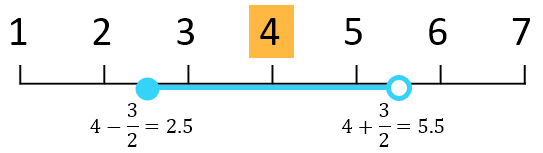`window = 4`

Current sample point = 4

2, 3, 4, 5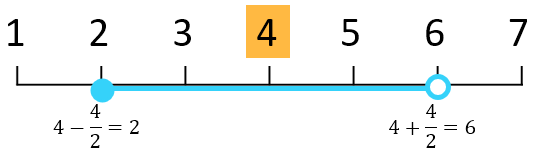For a vector window size, the leading edge and the trailing edge are included.

`window = [2 2]`

Current sample point = 4

2, 3, 4, 5, 6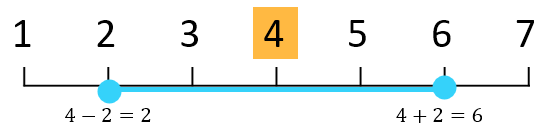For sample points near the endpoints of the input data, these moving statistic smoothing methods truncate the window so it begins at the first sample point or ends at the last sample point.

• `"movmean"`

• `"movmedian"`

• `"gaussian"`

`window = [2 2]`

Current sample point = 2

1, 2, 3, 4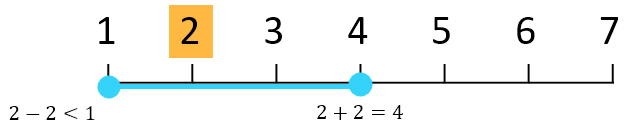For sample points near the endpoints of the input data, these local regression smoothing methods shift the window to include the first or last sample point.

• `"lowess"`

• `"lowess"`

• `"rlowess"`

• `"rloess"`

• `"sgolay"`

`window = [2 2]`

Current sample point = 2

1, 2, 3, 4, 5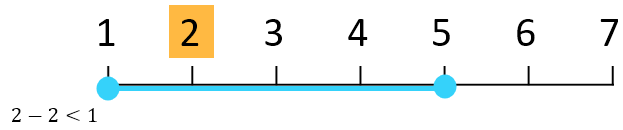## Algorithms

When the window size for the smoothing method is not specified, `smoothdata` computes a default window size based on a heuristic. For a smoothing factor τ, the heuristic estimates a moving average window size that attenuates approximately 100*τ percent of the energy of the input data.

## Version History

Introduced in R2017a

expand all